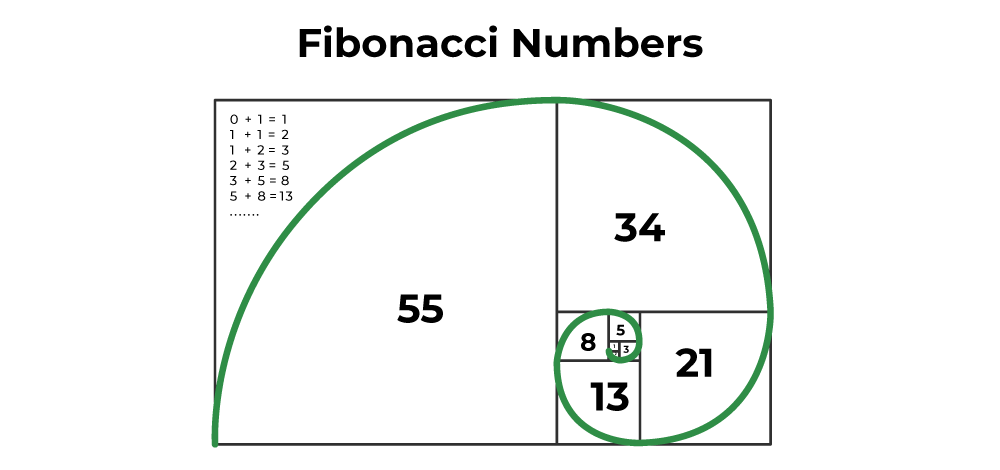GeeksforGeeks App
Open AppBrowser
Continue

# C Program to Print Fibonacci Series

In this article, we will discuss the Fibonacci series in C and the programs to print the Fibonacci series using recursion or loops in C.

## What is Fibonacci Series?

The Fibonacci series is the sequence where each number is the sum of the previous two numbers in the sequence. The first two numbers of the Fibonacci series are 0 and 1 and are used to generate the Fibonacci series.

In mathematical terms, the number at nth position can be represented by:

`Fn = Fn-1 + Fn-2`

with seed values

`F0 = 0 and F1 = 1`The Fibonacci programs in C that print the first n terms of the series can be coded using two methods specified below:

• Program to display the Fibonacci series in C using recursion.
• Program to display the Fibonacci series in C using loops.

## 1. Fibonacci Series using Recursion in  C

In this method, we will use a function that prints the first two terms and the rest of the terms are then handled by the other function that makes use of a recursive technique to print the next terms of the sequence.

## C

 `// C Program to print the Fibonacci series using recursion``#include ` `// first two values``int` `prev1 = 1;``int` `prev2 = 0;` `// recursive function to print the fibonacci series``void` `fib(``int` `n)``{``    ``if` `(n < 3) {``        ``return``;``    ``}``    ``int` `fn = prev1 + prev2;``    ``prev2 = prev1;``    ``prev1 = fn;``    ``printf``(``"%d "``, fn);``    ``return` `fib(n - 1);``}` `// function that handles the first two terms and calls the``// recursive function``void` `printFib(``int` `n)``{``    ``// when the number of terms is less than 1``    ``if` `(n < 1) {``        ``printf``(``"Invalid number of terms\n"``);``    ``}``    ``// when the number of terms is 1``    ``else` `if` `(n == 1) {``        ``printf``(``"%d "``, 0);``    ``}``    ``// when the number of terms is 2``    ``else` `if` `(n == 2) {``        ``printf``(``"%d %d"``, 0, 1);``    ``}``    ``// number of terms greater than 2``    ``else` `{``        ``printf``(``"%d %d "``, 0, 1);``        ``fib(n);``    ``}``    ``return``;``}` `// driver code``int` `main()``{``    ``int` `n = 9;``    ``printFib(n);``    ``return` `0;``}`

Output

`0 1 1 2 3 5 8 13 21 `

### Time Complexity

The time complexity of the program to print the first n terms of fibonacci series using recursion is O(n).

It is because for printing n terms,  the fib() function will call itself recursively for (n – 2) times and each time it will take constant time.

### Space Complexity

The space complexity of the above program is O(n)

It is because, for each recursive call of the fib() function, a separate stack frame is created. For (n-2) calls, (n-2) stack frames are created with results in the O(n) space complexity.

## 2. Fibonacci Series using Loops in C

In this method, we use one of the C loops to iterate and print the current term.F1 and F2 are handled separately. After that, we use two variables to store the previous and previous terms and keep updating them as we move to print the next term.

## C

 `// C Program to print the fibonacci series using iteration``// (loops)``#include ` `// function to print fibonacci series``void` `printFib(``int` `n)``{``    ``if` `(n < 1) {``        ``printf``(``"Invalid Number of terms\n"``);``        ``return``;``    ``}` `    ``// when number of terms is greater than 0``    ``int` `prev1 = 1;``    ``int` `prev2 = 0;` `    ``// for loop to print fibonacci series``    ``for` `(``int` `i = 1; i <= n; i++) {``        ``if` `(i > 2) {``            ``int` `num = prev1 + prev2;``            ``prev2 = prev1;``            ``prev1 = num;``            ``printf``(``"%d "``, num);``        ``}` `        ``// for first two terms``        ``if` `(i == 1) {``            ``printf``(``"%d "``, prev2);``        ``}``        ``if` `(i == 2) {``            ``printf``(``"%d "``, prev1);``        ``}``    ``}``}` `// driver code``int` `main()``{` `    ``int` `n = 9;``    ``printFib(n);``    ``return` `0;``}`

Output

`0 1 1 2 3 5 8 13 21 `

### Time Complexity

The time complexity of the program to print Fibonacci series up to n terms using loops is O(n). Because for n number for terms, the loop inside the printFib() function will be executed n times.

### Space Complexity

The space complexity of the above program is O(1). Because we only used a few variables which don’t depends on the number of terms to be printed.

My Personal Notes arrow_drop_up